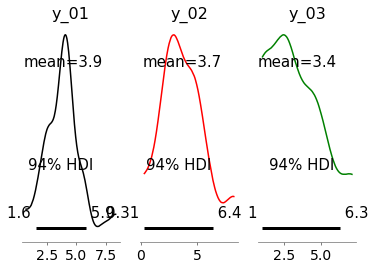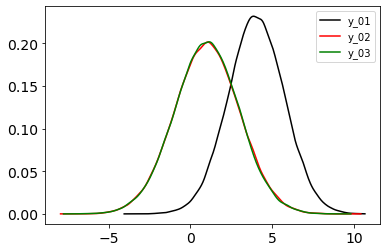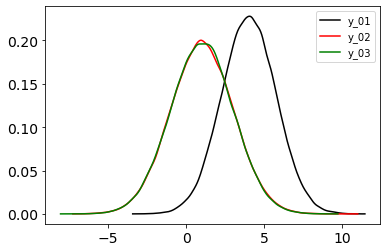# Comparison between do-operator and conditional inference

Hi all,

I am playing around with the do-operator while following along with the lecture Causality: Jonas Peters, Part 1 and the post by inFERENCe toy example.

models
``````def model_01():
x = numpyro.sample("x", dist.Normal(0, 1))
_y = numpyro.sample("_y", dist.Normal(0, 1))
y = numpyro.deterministic("y", x + 1 + jnp.sqrt(3) * _y)
return x, y

def model_02():
_y = numpyro.sample("_y", dist.Normal(0, 1))
_x = numpyro.sample("_x", dist.Normal(0, 1))
y = numpyro.deterministic("y", 1 + 2 * _y)
x = numpyro.deterministic("x", (y - 1) / 4 + jnp.sqrt(3) * _x / 2)
return x, y

def model_03():
_z = numpyro.sample("_z", dist.Normal(0, 1))
_y = numpyro.sample("_y", dist.Normal(0, 1))
y = numpyro.deterministic("y", _z + 1 + jnp.sqrt(3) * _y)
x = numpyro.deterministic("x", _z)
return x, y
``````

Given the three models above, I can see that both the joint distribution and the conditional, P(Y | X = 3) are equal.

With this toy example, I wanted to see how `numpyro.handlers.do` and `numpyro.handlers.condition` differ expecting the intervention P_{do(X = 3)} \ne P(Y | X = 3). As in the blog post, I can show that P_{do(X = 3)} \ne P(Y | X = 3) is true by comparing the between the Figure 1 and 2, posterior of `y`. The intervention and conditioning differ because each model have different structural causal equations.Figure 1: P(Y|X=3) for each model (the distributions look off because of the low number of samples. I am just trying to be consistent with the blog post)Figure 2: P_{do(X = 3)} for each model.Figure 3: `numpyro.handlers.condition(..., data={"x": 3})` for each model.

What surprises me is that `numpyro.handlers.do` and `numpyro.handlers.condition` result in the same posterior for `y`. Is this expected? I don’t think an intervention with a `do-operator` is the same as Bayesian inference so is this result expected because of the mechanism/implementation of `condition` and `do`?

2. At times I am using `numpyro.handlers.condition` on a deterministic site which I think may cause some issues, see this post.
I believe your second explanation is correct: `numpyro.handlers.condition` is not compatible with `numpyro.deterministic`. Currently it has no effect, but perhaps `condition` should be modified to raise a `NotImplementedError` for `deterministic` sites.